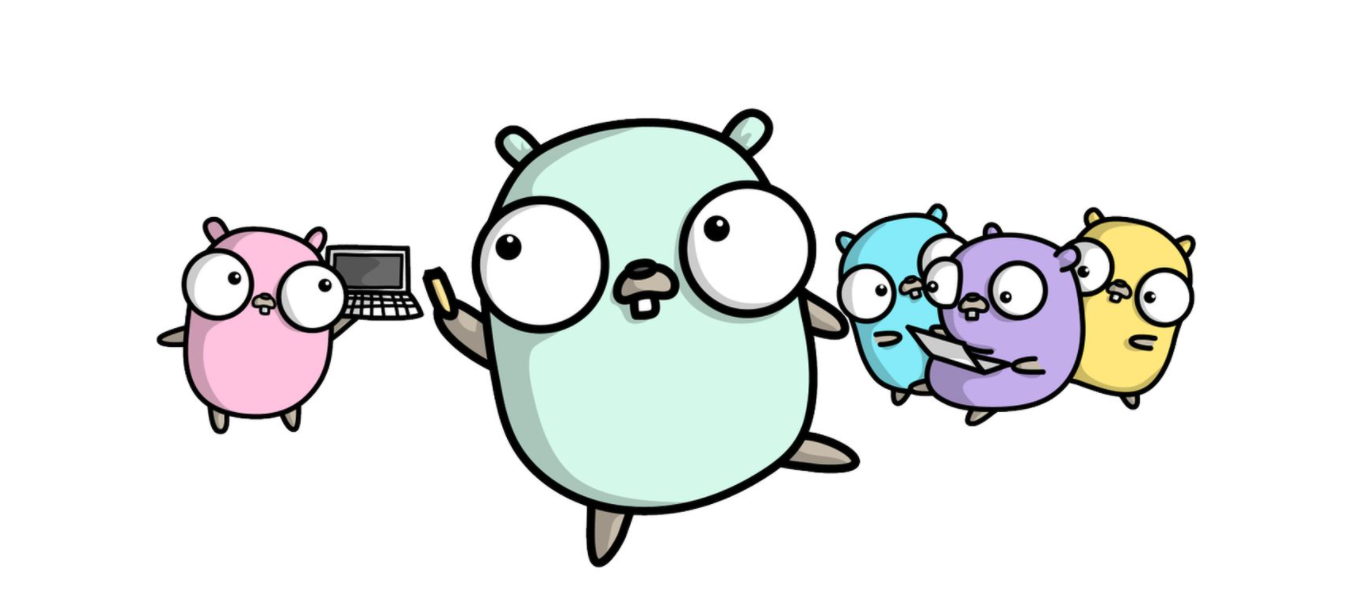# Go 语言，语法糖规则，可别掉入陷阱

• 2021 年 11 月 17 日
• 本文字数：779 字

阅读完需：约 3 分钟# 简短变量声明 :=

## 答题热身

func main() { i := 0 i, j := 1, 2 fmt.Printf("i = %d, j = %d", i, j)}

func main() { i, j := 0, 0 if true {  j, k := 1, 1  fmt.Printf("j = %d, k = %d\n", j, k) } fmt.Printf("i = %d, j = %d\n", i, j)}

1. 下面程序为什么不能通过编译

func test(i int) {  i := 0  fmt.Println(i)}

#### 答案：

i = 1, j = 2

j = 1, k = 1i = 0, j = 0

## 多变量赋值可能会重新声明

i, j := 0, 0j, k := 1, 1

• 当 := 左侧存在新的变量时（如 k）,那么已经声明的变量（如 j）会被重新声明。这并没有引入新的变量，只是把变量的值改变了。

• 当 := 左侧没有新变量编译报错。

## 不能用于函数外部

• := 这种简短变量声明只能用于函数中，用来初始化全局变量是不行的。

• 可以理解成 := 会拆分成两个语句，即声明和赋值。赋值语句不能出现在函数外部的。

# 可变参数 ...

• 可变参数必须在函数参数列表的最后一个（否则会引起编译时歧义）；

• 可变参数在函数内部是作为切片来解析的；

• 可变参数可以不填，不填时函数内部当成 nil 切片处理；

• 可变参数可以填入切片；

• 可变参数必须是相同类型的（如果需要是不同类型的可以定义为 interface{}类型）；

func sum(a int, vals...int) int {    total := a    for _, val := range vals {        total += val    }    return total}

## 不传值

fmt.Println(sum(1))          // "1"

## 传递多个参数

fmt.Println(sum(1, 2, 3, 4, 5)) // "15"

## 传递切片

values := []int{1, 2, 3, 4, 5}fmt.Println(sum(1, values...)) // "16"## 评论